# How to Subtotal Values for Groups and Only Keep One Subtotal for A Group in Column

Sometimes we may meet the case that subtotal values for different groups and only record a subtotal value for one group in a column, for other cells in this group, keep them blank. Today we will introduce you how can we subtotal values for groups and record subtotal values only in specific cells.

We can get subtotal through Subtotal function or SUM function in simple cases, but per different requirement, we need to apply different functions or combinations. Today we will provide a formula contains three functions IF/COUNTIF/SUMIFS to solve this issue. Through demonstrate a simple instance, we will introduce you the syntax, arguments of these functions, and let you know how the formula works step by step. After reading the article, you can think about if there are some other ways to solve this problem.

## EXAMPLE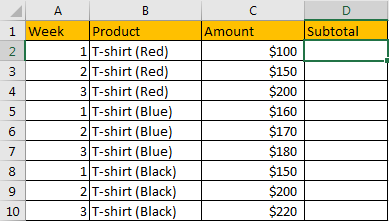Refer to above table, we can see that T-shirts are grouped by colors, and amounts for weeks are separately listed. Our expectation is “get subtotal for each product and record them in proper cell”, see example below: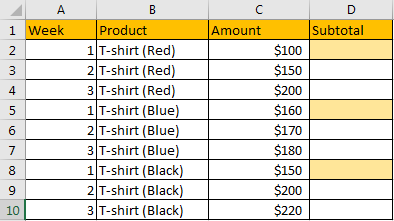We want to 1) save the subtotal values in the cells which background is filled in light orange. 2) For the other cells in this column should keep blank. 3) By the way, we also want to enter only one formula into D2, then though dragging the handle down to fill other cells, subtotal in D5 and D8 should also be calculated properly.

Can we create a formula that can cover above three conditions? Actually, yes.

In this instance, with the help of IF, COUNTIF and SUMIF functions, we can calculate subtotal for each product by only one formula properly.

## FORMULA

Step 1: Select B2:B10, then in Name Box define a new name for this range, for example ‘Product’.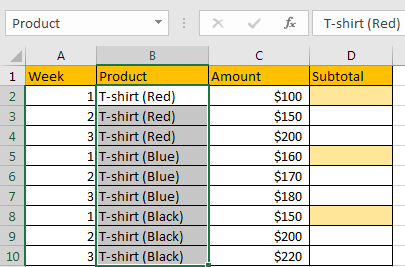Step 2: Select C2:C10, in Name Box define a new name for this range, for example ‘Amount’.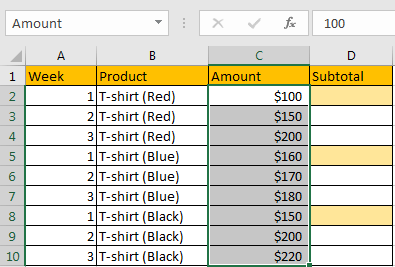Step 3: In D2, enter the formula =IF(COUNTIF(\$B\$2:B2,B2)=1,SUMIF(\$B\$2:\$B\$10,B2,\$C\$2:\$C\$10),””).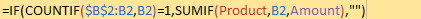NOTE: In step#1 and step#2 we defined “Product” and “Amount” two ranges, when entering the formula, after typing “Amou…”, defined range “Amount” is auto loaded. User defined range can be seen as absolute reference, so when copy formula to other cells, the reference will not be adjusted automatically. If we use range reference B2:B10 in the formula, we need to add “\$” to lock the range.

Step 4: Press Enter after typing the formula. A number is returned.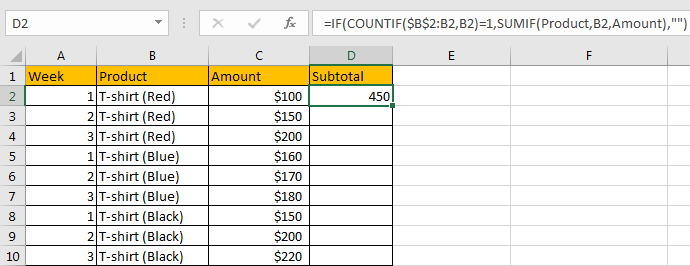We can see in column D2, subtotal \$450 is returned, it is equal to 100+150+200, the total of C2:C4. The formula is correct.

Step 5: Drag the handle down to fill other cells.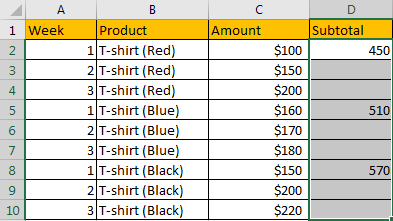Verify that subtotal values are calculated correctly for each product. Besides, the subtotal is only recorded in the first row of each group, others keep blank.

## FUNCTION INTRODUCTION

In this formula we create a formula with IF function which applies COUNTIF and SUMIF functions as its arguments.

IF function will run a logical test first, and based on the result ‘True’ of ‘False’, it chooses to which branch to execute.

For IF function, the syntax is:

=IF (logical_test, [value_if_true], [value_if_false])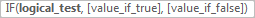———————————————————————————————-

COUNTIF function can used for counting cell number based on different criteria.

For COUNTIF function, the syntax is:

=COUNTIF (range, criteria)

———————————————————————————————-

SUMIFS function can be seen as SUM+IFS, it can handle multiple ‘criteria range’ and ‘criteria’ combinations.

For SUMIF function, the syntax is:

=SUMIF (range, criteria, [sum_range])

For above three functions, they all allow logical operators like “>”,”>”,”<>” and wildcards like “*”,”?”.

## FUNCTION ARGUMENTS EXPLANATION

For If function:

Logical_test: COUNTIF(\$B\$2:B2,B2)=1

value_if_true: SUMIF(\$B\$2:\$B\$10,B2,\$C\$2:\$C\$10)

value_if_false: “” – nothing returns

——————————————————————————————————————–

For COUNTIF function:

Range: \$B\$2:B2

Criteria: B2

For range \$B\$2:B2 is an expanding range. Start cell is B2, and the end cell is not fixed.

Select range and criteria separately in the formula bar, and press F9 to convert cell reference to real values. See screenshot below: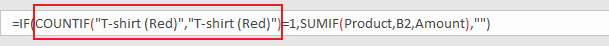——————————————————————————————————————–

For SUMIF function:

Range: \$B\$2:\$B\$10

Criteria: B2

Sum Range: \$C\$2:\$C\$10

Select above arguments, press F9 to convert cell reference to real values. See screenshot below: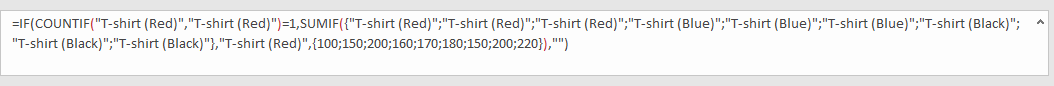## HOW THIS FORMULA WORKS

After explaining each argument in the formula, now we will show you how the formula works with these arguments.

Refer to above steps, the formula is converted to below format in the formula bar.

`=IF(COUNTIF("T-shirt (Red)","T-shirt (Red)")=1,SUMIF({"T-shirt (Red)";"T-shirt (Red)";"T-shirt (Red)";"T-shirt (Blue)";"T-shirt (Blue)";"T-shirt (Blue)";"T-shirt (Black)";"T-shirt (Black)";"T-shirt (Black)"},"T-shirt (Red)",{100;150;200;160;170;180;150;200;220}),"")`

——————————————————————————————————————–

In IF function, the core part is logical test part, its returned value determines which branch we will go to.

Logical_test:

`COUNTIF("T-shirt (Red)","T-shirt (Red)")=1`

For this part, range “T-shirt (Red)” just contains one criteria “T-shirt (Red)”, so COUNTIF(“T-shirt (Red)”,”T-shirt (Red)”)=1, the formula COUNTIF(“T-shirt (Red)”,”T-shirt (Red)”)=1 is ‘True’. So we go to ‘value_if_ture’ and ignore ‘value_if_false’.

value_if_true:

`SUMIF({"T-shirt (Red)";"T-shirt (Red)";"T-shirt (Red)";"T-shirt (Blue)";"T-shirt (Blue)";"T-shirt (Blue)";"T-shirt (Black)";"T-shirt (Black)";"T-shirt (Black)"},"T-shirt (Red)",{100;150;200;160;170;180;150;200;220})`

For this part, we have one pair of criteria range and criteria:

criteria range:

`{"T-shirt (Red)";"T-shirt (Red)";"T-shirt (Red)";"T-shirt (Blue)";"T-shirt (Blue)";"T-shirt (Blue)";"T-shirt (Black)";"T-shirt (Black)}`

criteria:

`"T-shirt (Red)"`

Compare each value in the array with criteria, we get a new array that only contains ‘True’ and ‘False’.

`{True;True;True;False;False;False;False;False}`

Convert True to 1, False to 0.

{1;1;1;0;0;0;0;0}

Now, we have below two arrays in the formula:

Sum Range:

`{100;150;200;160;170;180;150;200;220}`

{1;1;1;0;0;0;0;0}

We list the two arrays in two rows, multiply the two numbers in the same column, and save their products in another row, sum all products together, we can get 450 at last.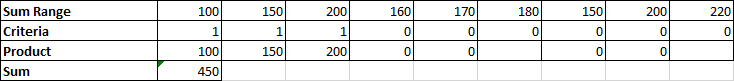## NOTE

We noticed that after typing the formula and dragging the handle down to fill the ‘Subtotal’ column, not all the cells have a sum value, some cells keep blank as we expect. Why the formula returns an empty value after coping it to other cells?

In cell D3, the formula is automatically adjusted to =IF(COUNTIF(\$B\$2:B3,B3)=1,SUMIF(Product,B3,Amount),””) after coping and pasting.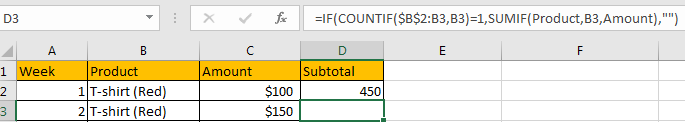In logical test part, CONTIF(\$B\$2:B3,B3) is equal to COUNTIF({“T-shirt (Red)”;”T-shirt (Red)”},”T-shirt (Red)”).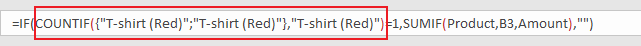Obviously, this formula returns 2, which is not equal to 1, so IF function returns ‘valus_if_false’ value, which is “” an empty value in this case. Above all, in D3, the subtotal is blank.

## SUMMARY

1. To subtotal values we can apply different functions together. In this case we apply IF function core formula, and a formula contains COUNTIF function as logical test, SUMIFS function as ‘True’ part returned value.

2. For above three functions, they all allow logical operators like “>”,”>”,”<>” and wildcards like “*”,”?”.

### Related Functions

• Excel IF function
The Excel IF function perform a logical test to return one value if the condition is TRUE and return another value if the condition is FALSE. The IF function is a build-in function in Microsoft Excel and it is categorized as a Logical Function.The syntax of the IF function is as below:= IF (condition, [true_value], [false_value])….
• Excel COUNTIF function
The Excel COUNTIF function will count the number of cells in a range that meet a given criteria. This function can be used to count the different kinds of cells with number, date, text values, blank, non-blanks, or containing specific characters.etc.= COUNTIF (range, criteria)…
• Excel SUMIF Function
The Excel SUMIF function sum the numbers in the range of cells that meet a single criteria that you specify. The syntax of the SUMIF function is as below:=SUMIF (range, criteria, [sum_range])…
• Excel SUMIFS Function
The Excel SUMIFS function sum the numbers in the range of cells that meet a single or multiple criteria that you specify. The syntax of the SUMIFS function is as below:=SUMIFS (sum_range, criteria_range1, criteria1, [criteria_range2, criteria2], …)…
• Excel SUM function
The Excel SUM function will adds all numbers in a range of cells and returns the sum of these values. You can add individual values, cell references or ranges in excel.The syntax of the SUM function is as below:= SUM(number1,[number2],…)
• Excel SUBTOTAL function
The Excel SUBTOTAL function returns the subtotal of the numbers in a list or database. The syntax of the SUBTOTAL function is as below:= SUBTOTAL (function_num, ref1, [ref2])….